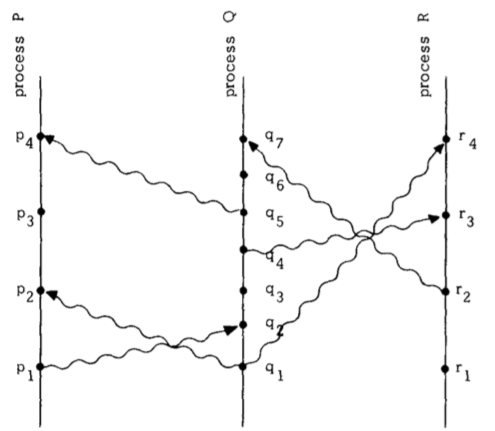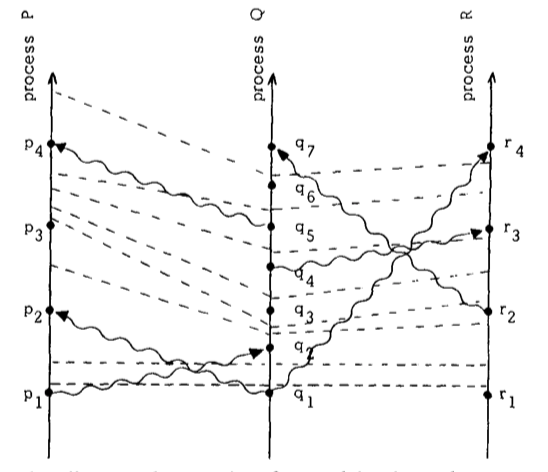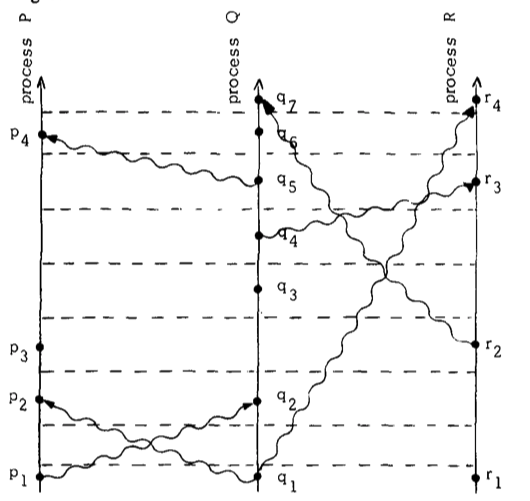# 【考古】【必读】分布式理论奠基 paper

Posted 2021-04-06 21:31:59 ‐ 30 min read

## The Partial Ordering

1. 如果$a$和$b$是同一个进程中的事件，而且$a$发生于$b$之前，那么$a \rightarrow b$
2. 如果$a$是一个进程中发送消息，$b$是另一个进程中接收消息，那么$a \rightarrow b$
3. 如果$a \rightarrow b$并且$b \rightarrow c$，那么$a \rightarrow c$$a \rightarrow b$另一个定义是事件$a$可以影响到$b$。两个事件如果不能互相影响就是并发的。即使我们在图中看出$q_3$在物理时间上早于$p_3$，进程 P 不知道进程 Q 上发生了$q_3$事件，直到$p_4$收到消息（在$p_4$之前，P 进程最多直到 Q 进程将要发生事件$q_3$）

This definition will appear quit natural to the reader familiar with the invariant space-time formulation of special relativity, as described for example in  or the first chapter of . In relativity, the ordering of events is defined in terms of messages that could be sent. However, we have taken the more pragmatic approach of only considering messages that actually are sent. We should be able to determine if a system performed correctly by knowing only those events which did occur, without knowing which events could have occurred.【这一段不知道怎么翻译。】

## Logical Clocks

Clock Condition：对于任意事件$a, b$，如果 $a \rightarrow b$，则$C\langle a \rangle \lt C\langle b \rangle$。

Clock Condtion（$\rightarrow$）满足如下条件：

1. 如果事件$a,b$都是进程$P_i$的，$a$早于$b$，那么$C_i\langle a \rangle \lt C_i\langle b \rangle$。
2. 如果事件$a$是进程$P_i$发送消息，$b$是进程$P_j$接收消息，那么$C_i\langle a \rangle \lt C_j\langle b \rangle$IR1. 进程$P_i$在两个连续事件之间的$C_i$增长

IR2. 1. 如果事件$a$是进程$P_i$发送消息$m$，消息$m$包含时间戳$T_m = C_i\langle a \rangle$ 2. 收到消息$m$的进程$P_j$设置$C_j$大于等于$T_m$

## Ordering the Events Totally

$\Rightarrow$依赖于系统时钟，不是唯一的，不同的时钟选择会导致不同的$\Rightarrow$，而偏序关系$\rightarrow$由系统的事件唯一确定。

I. 一个已经获取这一资源的进程在另一进程获取前释放该资源 II. 不同进程的资源授权必须按照申请资源的顺序授权 III. 如果每个被授权的进程都释放了资源，那么每个请求都被处理了

1. 为了请求资源，进程$P_i$发送消息$T_m$：$P_i$向其他所有进程请求资源，将消息放入自己的请求队列，$T_m$是消息的时间戳
2. 当进程$P_j$收到消息$T_m$，也将其放入自己的消息队列，回$P_i$一个 ack
3. 为了释放资源，进程$P_i$从消息队列删除消息$T_m$，然后发送一个$P_i$释放资源的消息给其他所有进程
4. 当进程$P_j$收到$P_i$释放资源的消息，也将其$T_m$从自己的消息队列删除
5. 进程$P_i$满足下面两个条件时被授权资源：(1). 消息队列中有$T_m$ (2) 收到了其他所有的进程的消息晚于$T_m$（注意到这两个条件只需要$P_i$自己就可以确定）

## Anomalous Behavior

Strong Clock Condition。对于$\varphi$中的任意事件$a,b$，如果$a \rightarrow b$, 则 $C\langle a \rangle < C\langle b \rangle$

## Physical Clocks

PC1. 存在一个常数 $\kappa \ll 1$，对于所有的$i$: $|dC_i(t)/dt - 1| \lt \kappa$。$\kappa$的典型值为$10^{-6}$

PC2. 对于所有的$i,j$，$|C_i(t) - C_j(t)| < \epsilon$

IR1' 对于每个$i$，如果$P_i$在物理时间$t$没有收到消息，则$C_i$在$t$是可微的，并且$dC_i(t)/dt > 0$

IR2' (a) 如果$P_i$在时间$t$发送了消息$m$，$m$中包含了时间戳$T_m = C_i(t)$ (b) 在进程$P_j$在物理时间$t'$收到消息$m$，将$C_j(t')$设置为$max(C_j(t' - 0), T_m + \mu_m)$

【译者注：】通过一个数理公式证明了满足 PC2，这里省去翻译，数理公式的证明在 Appendix，同样略去

## 参考文章：

1. https://lrita.github.io/2018/10/24/lamport-logical-clocks-vector-lock/
2. 狭义相对论与分布式系统中的时间 https://www.jianshu.com/p/0c79d650d13f
1. 阐述了分布式系统中时间的本质，探索了分布式理论的本质
2. 提出了 Logical Clock 算法，是后续 Vector Clock，HLC（混合逻辑时钟，包含了 logic clock, physical clock) 等的基础
3. 提出了 Replicated State Machine 的理念，是后续 Paxos 及其应用的基础
4. 设计了无中心的分布式临界资源算法，是后续多种无中心分布式算法的鼻祖
5. 设计了时间同步的雏形算法，后续 NTP 等的基础
3. 阿里数据库的 HLC https://database.51cto.com/art/201911/606198.htm
4. https://lrita.github.io/2018/10/19/communication-model-in-distribution/
5. http://zhangtielei.com/posts/blog-distributed-causal-consistency.html 【推荐】
6. 分布式事务中的时间戳 【推荐】

## 更新记录

• 2020-4-21 更新，加入参考文章【分布式事务中的时间戳】